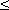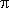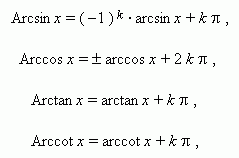# Inverse trigonometric functions

Inverse trigonometric functions. Multiple-valued functions.
Principal values of inverse trigonometric functions.

The relation x = sin y permits to find both x by the given y , and also y by the given x ( at  | x |1 ). So, it is possible to consider not only a sine as a function of an angle, but an angle as a function of a sine. The last fact can be written as: y = arcsin x ( “arcsin” is read as “arcsine” ). For instance, instead of 1/2 = sin 30° it is possible to write: 30° = arcsin 1/2. At the second record form an angle is usually represented in a radian measure:/ 6 = arcsin 1/2.

Definitions. arcsin x is an angle, a sine of which is equal to x . Analogously the functions arccos x , arctan x , arccot x , arcsec x , arccosec x are defined. These functions are inverse to the functions  sin x ,  cos x ,  tan x ,  cot x ,  sec x ,  cosec x ,  therefore they are called inverse trigonometric functions. All inverse trigonometric functions are multiple-valued functions , that is to say for one value of argument an innumerable set of a function values is in accordance. So, for example, angles 30°, 150°, 390°, 510°, 750° have the same sine. A principal value of arcsin x is that its value, which is contained between  –/ 2 and  +/ 2  ( –90° and +90° ), including the bounds :/ 2arcsin x+/ 2 .

A principal value of arccos x is that its value, which is contained between  0  and( 0° and  +180° ), including the bounds :

0arccos x.

A principal value of arctan x is that its value, which is contained between –/ 2  and  +/ 2   ( –90° and +90° ) without the bounds :/ 2  <  arctan x <  +/ 2 .

A principal value of arccot x is that its value, which is contained between  0  and( 0° and  +180° ) without the bounds :

0  <  arccot x <.

If  to sign any of values of inverse trigonometric functions as Arcsin x , Arccos x , Arctan x , Arccot x and to save the designations: arcsin x , arcos x , arctan x , arccot x for their principal values, then there are the following relations between them:where k – any integer. At k = 0  we have principal values.